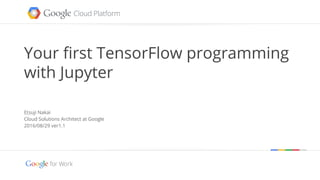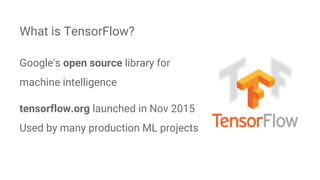Successfully reported this slideshow.

# Your first TensorFlow programming with Jupyter×

# Your first TensorFlow programming with Jupyter

「OSSユーザーのための勉強会 <oss>　#15 TensorFlow」での発表予定資料です。
http://eventregist.com/e/ossx2016-9

「OSSユーザーのための勉強会 <oss>　#15 TensorFlow」での発表予定資料です。
http://eventregist.com/e/ossx2016-9

### Your first TensorFlow programming with Jupyter

1. 1. Google confidential | Do not distribute Your first TensorFlow programming with Jupyter Etsuji Nakai Cloud Solutions Architect at Google 2016/08/29 ver1.1
2. 2. Etsuji Nakai Cloud Solutions Architect at Google The author of “Introduction to Machine Learning Theory” (Japanese Book) New book “ML programming with TensorFlow” will be published soon! \$ who am i
3. 3. Google's open source library for machine intelligence tensorflow.org launched in Nov 2015 Used by many production ML projects What is TensorFlow?
4. 4. Web based interactive data analysis platform. Can be used as a TensorFlow runtime environment. What is Jupyter? How to use Jupyter on GCP? (Japanese Blog) http://enakai00.hatenablog.com/entry/2016/07/03/201117
5. 5. ● All calculations are done in a “Session” ● The session contains: ○ Placeholders : where you put actual data ○ Variables : to be optimized by the algorithm ○ Functions : consisting of placeholders and variables ○ Training algorithm : to optimize the variables Programming Paradigm of TensorFlow
6. 6. Programming Paradigm of TensorFlow ● Three steps to write a program with TnesorFlow ○ Define a model with placeholders, variables, functions. ○ Define a loss function and a training algorithm. ○ Run session to optimize the variables minimizing the loss function.
7. 7. Example: Least Squares Method ● Figure out a smooth curve which predicts next year’s temperature. ● In matrix representation: Monthly average temperature in Tokyo. VariablePlaceholder Function
8. 8. ● Define a loss function ● In matrix representation: Example: Least Squares Method Placeholder Function Observed temperature Prediction vs Observed Values
9. 9. ● The matrix representations can be directly translated into TensorFlow codes. Example: Least Squares Method x = tf.placeholder(tf.float32, [None, 5]) w = tf.Variable(tf.zeros([5, 1])) y = tf.matmul(x, w) t = tf.placeholder(tf.float32, [None, 1]) loss = tf.reduce_sum(tf.square(y-t))
10. 10. ● Specify an optimization algorithm. ● Finally, prepare a session and run the optimization loop. Example: Least Squares Method sess = tf.Session() sess.run(tf.initialize_all_variables()) i = 0 for _ in range(100000): i += 1 sess.run(train_step, feed_dict={x:train_x, t:train_t}) if i % 10000 == 0: loss_val = sess.run(loss, feed_dict={x:train_x, t:train_t}) print ('Step: %d, Loss: %f' % (i, loss_val)) train_step = tf.train.AdamOptimizer().minimize(loss) Putting actual data values in placeholders
11. 11. ● You can see the actual result at: ○ http://goo.gl/Dojgp4 Example: Least Squares Method
12. 12. Demo: More Interesting Examples!
13. 13. Thank you!